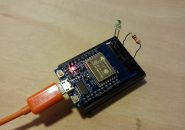## 在Arduino上使用传感器：创建一个可以检测运动并作出响应的简单设备<h3超声波传感器的工作原理 我们今天要了解的超声波传感器是HC-SR05, 它是旧版HC-SR04的5引脚升级版本。该设备具有两个圆柱形模块。一个是发出超声波信号的发射器，另一个是接收器，会捕获到达物体后反弹回来的信号。通过测量信号返回的时间，传感器可以计算出物体的距离。

### 所需组件

 Arduino UnoHC-SR05 超声波传感器 （可以使用HC-SR04或其他超声波传感器，但需要修改引脚配置）Arduino IDEUSB 电缆 如果您还没有设置Arduino IDE，可以先查阅我们之前发布的指南

### 代码

void setup() {
pinMode (6,OUTPUT); //Insert HC-SR-05 with VCC in pin 6
pinMode (5, INPUT); // Assign pin 5 to Echo
pinMode (4, OUTPUT);// Assign pin 4 to Trig
pinMode (2,OUTPUT); // Assign pin 2 to GND.
Serial.begin(9600); // This will allow you to read how far away your sensor is later
}
void loop()
{
long duration, cm; // Initialize variables for duration and cm
digitalWrite(6, HIGH); // Power the sensor
digitalWrite(4, LOW); // Clear pulse before sending a 10 microsecond ping,
delayMicroseconds(2);
digitalWrite(4, HIGH);
delayMicroseconds(10);
digitalWrite(4, LOW);
duration = pulseIn(5, HIGH); // Detect pulse length from the Echo pin, measured in microseconds
cm = (duration/2)/29.155; // Divide duration in half (due to round trip), then convert distance to centimeters (1cm per 29.155 microseconds), assign to cm variable
Serial.print(cm); // Print distance in cm to serial monitor
Serial.print(“cm”);
Serial.println();
delay(100); // Delay
int note = 0; // Assign note based on distance, spacing notes per 6cm
if(cm<=48&&cm>42) {
note = 523;
} else if(cm<=42&&cm>36) {
note = 493;
} else if(cm<=36&&cm>30) {
note = 440;
} else if(cm<=30&&cm>24) {
note = 392;
} else if(cm<=24&&cm>18) {
note = 349;
} else if(cm<=18&&cm>12) {
note = 329;
} else if(cm<=12&&cm>6) {
note = 294;
} else if(cm<=6) {
note = 261;
}
if (note == 0) { // If distance isn’t within 48cm, play nothing noTone(9);
} else {
tone(9, note, 200); // Play assigned note for 200 milliseconds
}
delay(10); // Brief pause
}

digitalWrite(6, HIGH); // Power the sensor
digitalWrite(4, LOW); // Clear pulse before sending a 10 microsecond ping,
delayMicroseconds(2);
digitalWrite(4, HIGH);
delayMicroseconds(10);
digitalWrite(4, LOW);
duration = pulseIn(5, HIGH); // Detect pulse length from the Echo pin, measured in microseconds
cm = (duration/2)/29.155; // Divide duration in half (due to round trip), then convert distance to centimeters (1cm per 29.155 microseconds), assign to cm variable

int note = 0; // Assign note based on distance, spacing notes per 6cm
if(cm<=48&&cm>42) {
note = 523;
} else if(cm<=42&&cm>36) {
note = 493;
} else if(cm<=36&&cm>30) {
note = 440;
} else if(cm<=30&&cm>24) {
note = 392;
} else if(cm<=24&&cm>18) {
note = 349;
} else if(cm<=18&&cm>12) {
note = 329;
} else if(cm<=12&&cm>6) {
note = 294;
} else if(cm<=6) { note = 261;
}
if (note == 0) { // If distance isn’t within 48cm, play nothing noTone(9);
} else {
tone(9, note, 200); // Play assigned note for 200 milliseconds
}### 接线

● 将HC-SR05传感器插入数字引脚2到6，GND插入引脚2，VCC插入引脚6。这样连接可以使传感器朝向外侧，远离Arduino。
● 将扬声器上的正极线（通常为红色）插入引脚9。
● 将另一根扬声器线（通常为黑色）插入其中一个GND引脚。 连接好所有电线好，插入Arduino，并在传感器前留出大约50厘米的空间。接下来是最有趣的部分。将您的手或者一根小棍子放在传感器前面，您会听到扬声器发出的声音。物体放置的越远，音调越高。### 相关文章

1.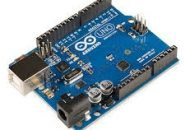#### 如何用调光器控制灯光

2.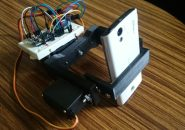3. #### 用Arduino Uno制作一个智能的自动宠物喂食器（续）

4.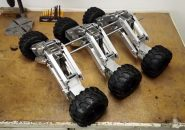5. #### 使用Arduino驱动LCD的DIY指南

6.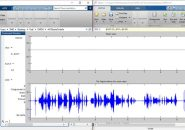#### 使用MATLAB进行数字信号处理-第2部分

7.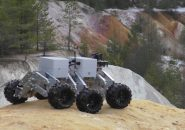#### Arduino探索漫游车3—编程

8.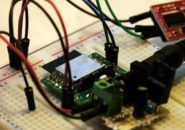#### ESP-WROOM-02 Wifi设置指南—AT指令

9.